# Inter 1st year Maths Differentiation solutions

Mathematics intermediate first year 1b differentiation chapter 9 solutions for some problems.

Here you can also see the solutions for inter maths 1a and 1b.

You can see the solutions for junior inter 1b

1. Locus

The straight line sa

Straight lines la

11. Rate measure

You can also see the solutions for junior inter maths 1A

You can see the solutions for text book Maths 1A

Functions

Exercise 1(a)

Exercise 1(b)

Exercise 1(c)

Mathematical Induction

Exercise 2(a)

Matrices

Exercise 3(a)

Exercise 3(b)

Exercise 3(c)

Exercise 3(d)

Exercise 3(e)

Exercise 3(f)

Exercise 3(g)

Exercise 3(h)

Exercise 3(i)

Exercise 4(a)

Exercise 4(b)

Product of vectors

Exercise 5(a)

Exercise 5(b)

Exercise 5(c)

Trigonometric Ratios up to Transformations

Exercise 6(a)

Exercise 6(b)

Exercise 6(c)

Exercise 6(d)

Exercise 6(e)

Exercise 6(f)

Trigonometric Equations

Exercise 7(a)

Inverse Trigonometric Equations

Exercise 8(a)

Hyperbolic Functions

Exercise 9(a)

Properties of Triangles

Exercise 10(a)

Exercise 10(b)

Maths 1B solutions for

Locus

Exercise 1(a)

Transformation of Axes

Exercise 2(a)

Direction Cosines and Direction Ratios

Exercise 6(a)
Exercise 6(b)
The Plane

Exercise 7(a)
Limits and Continuity

Exercise 8(a)

Exercise 8(b)

Exercise 8(e)

M

## Intermediate Mathematics 1b chapter 9 solutions

Differentiation

Solutions for very short answer questions.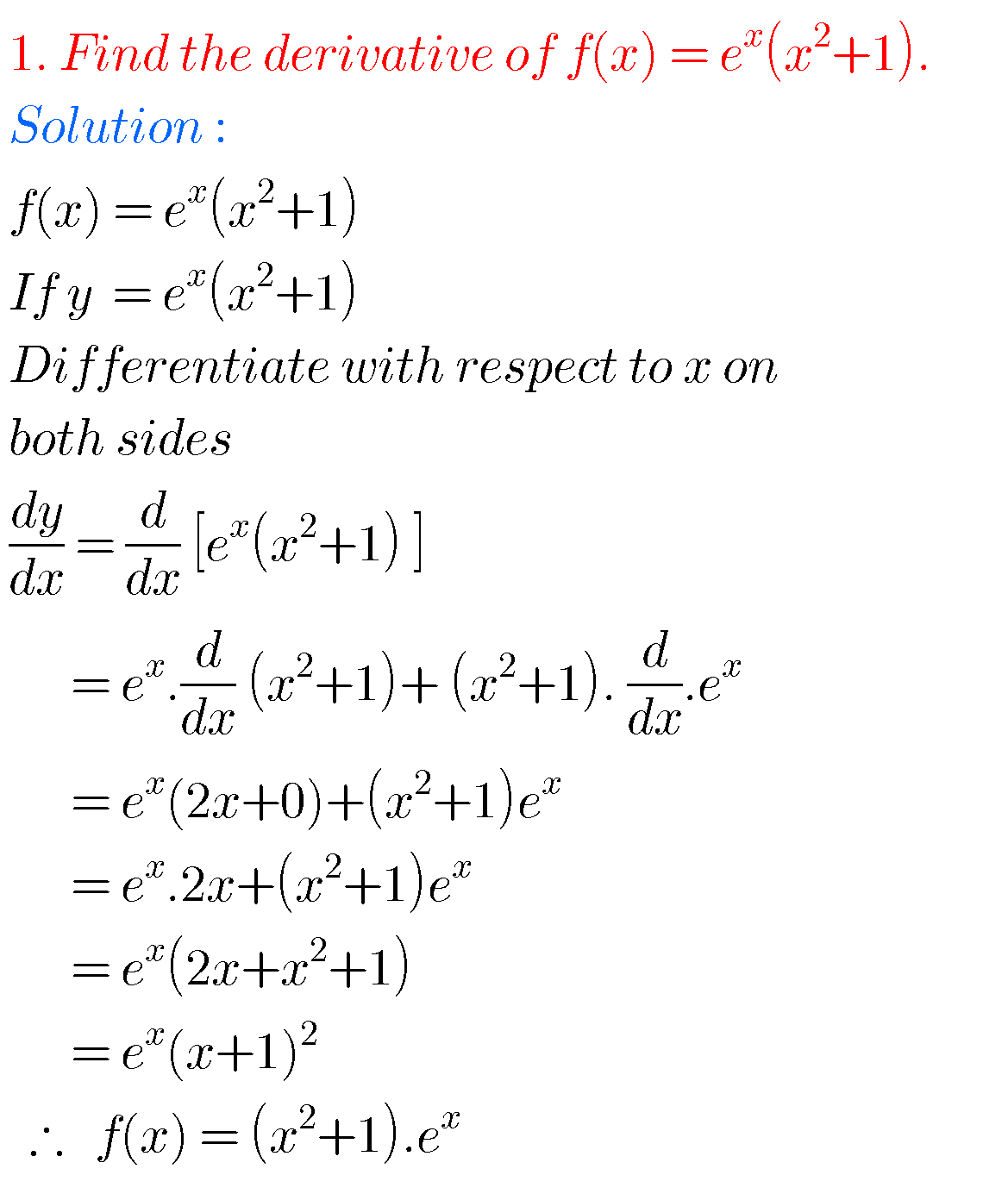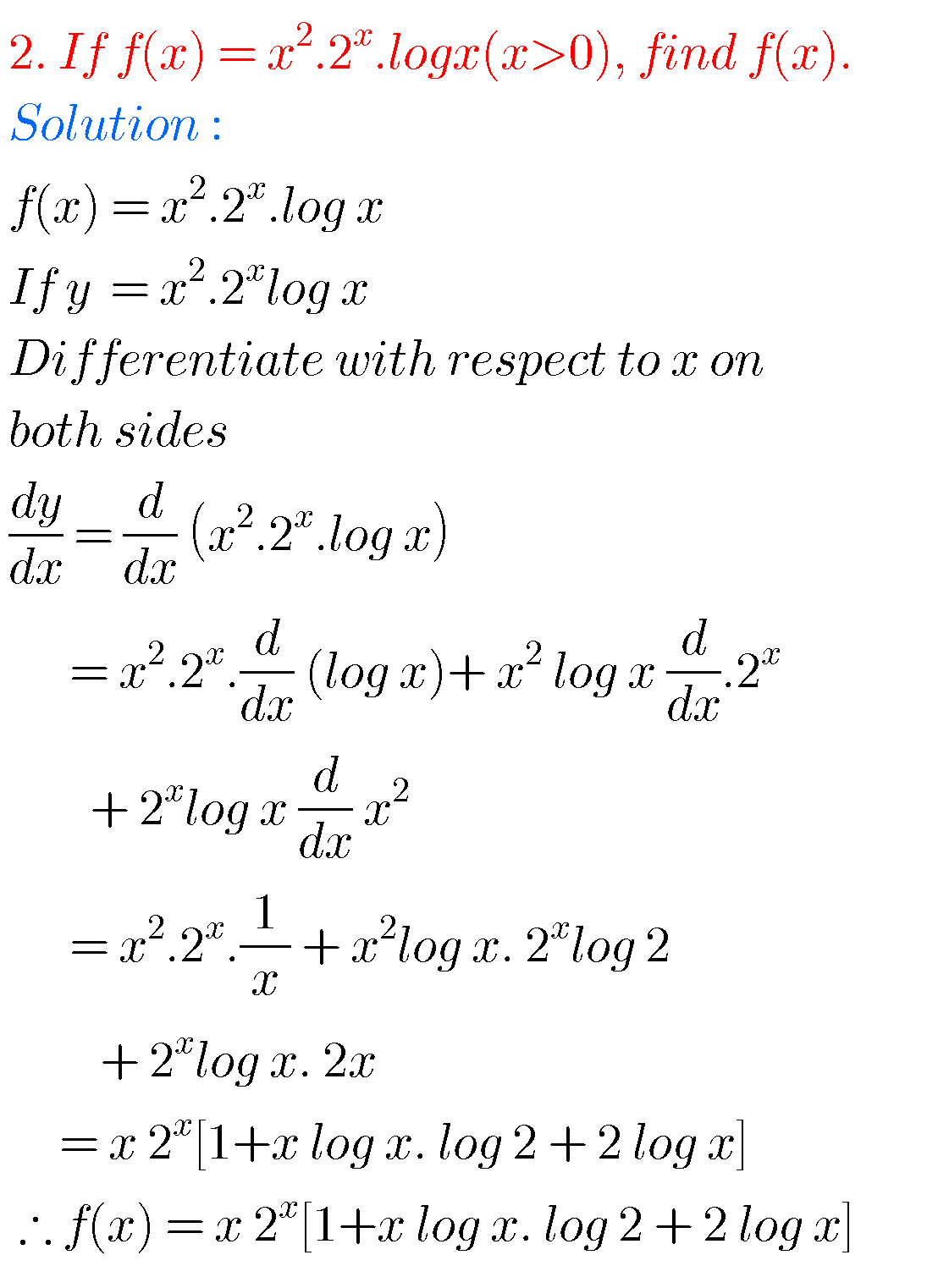###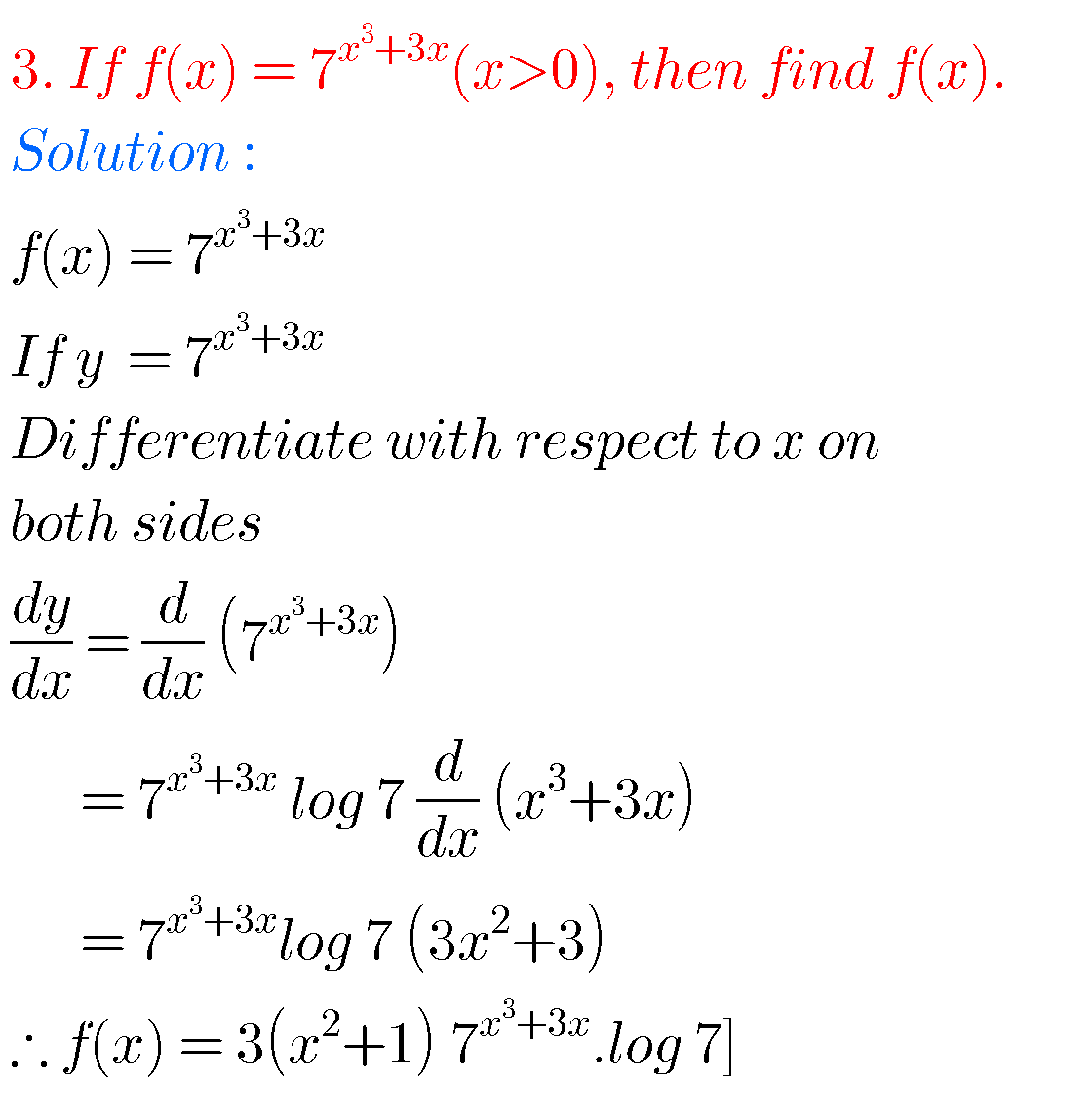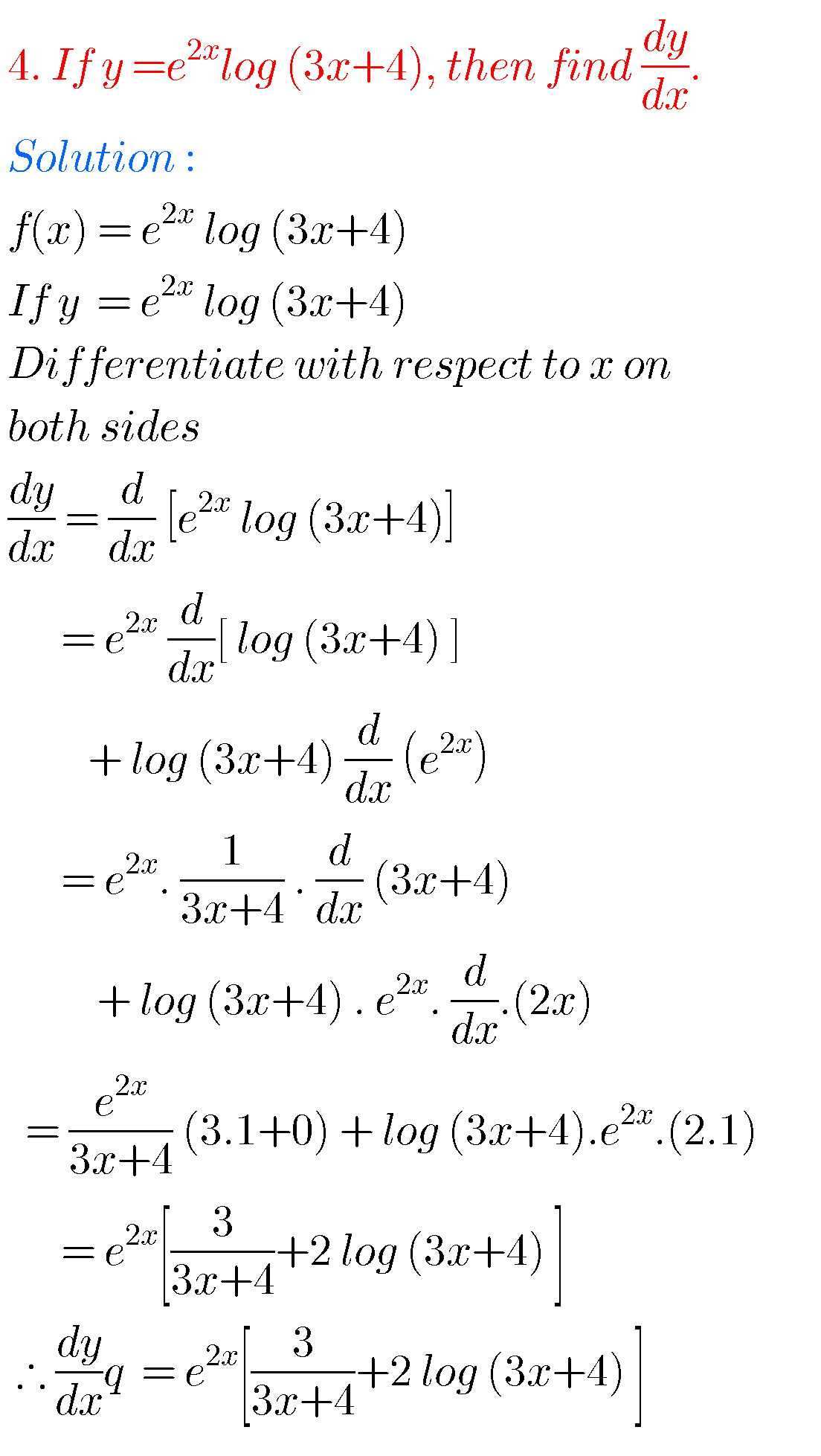###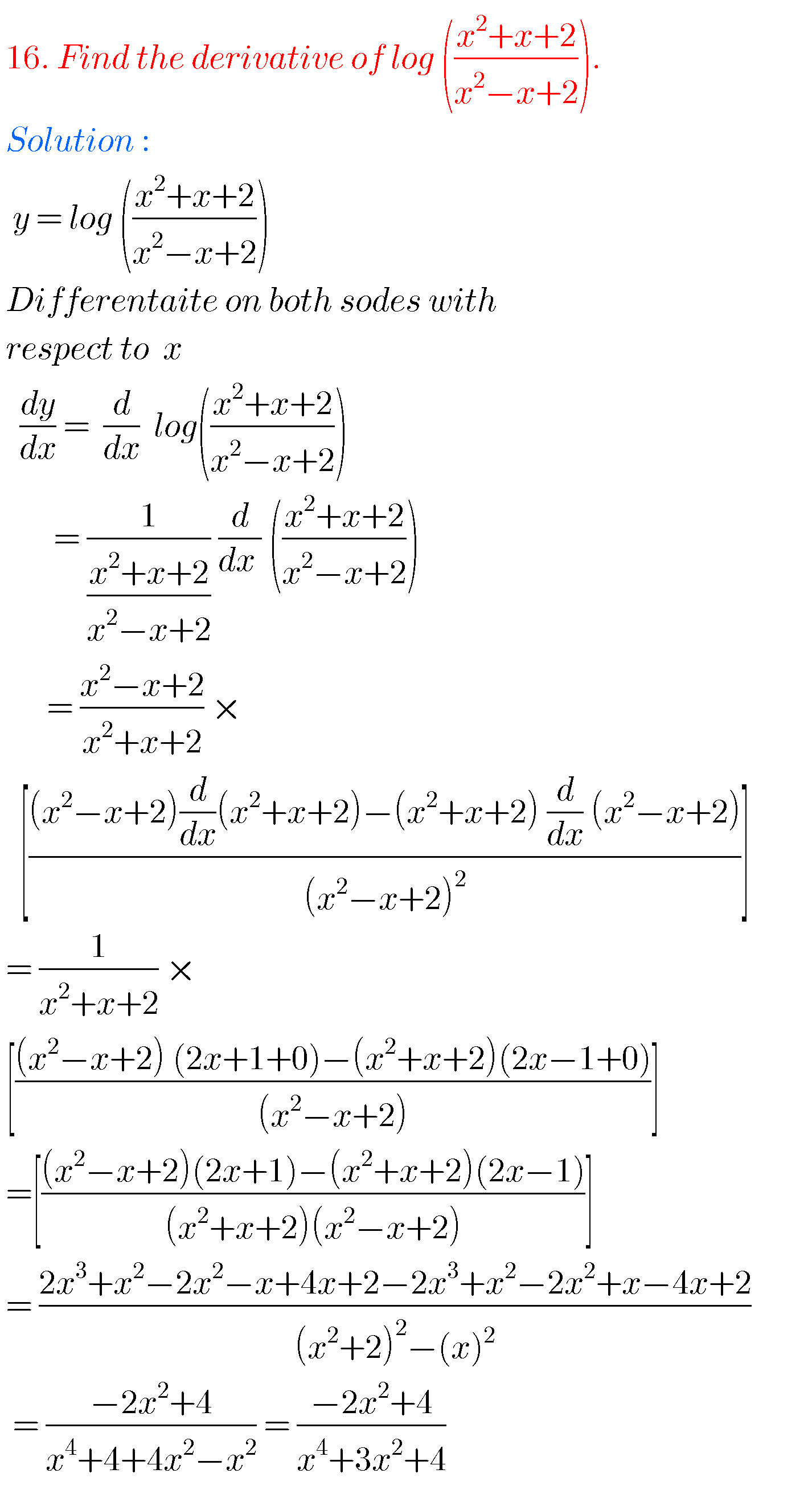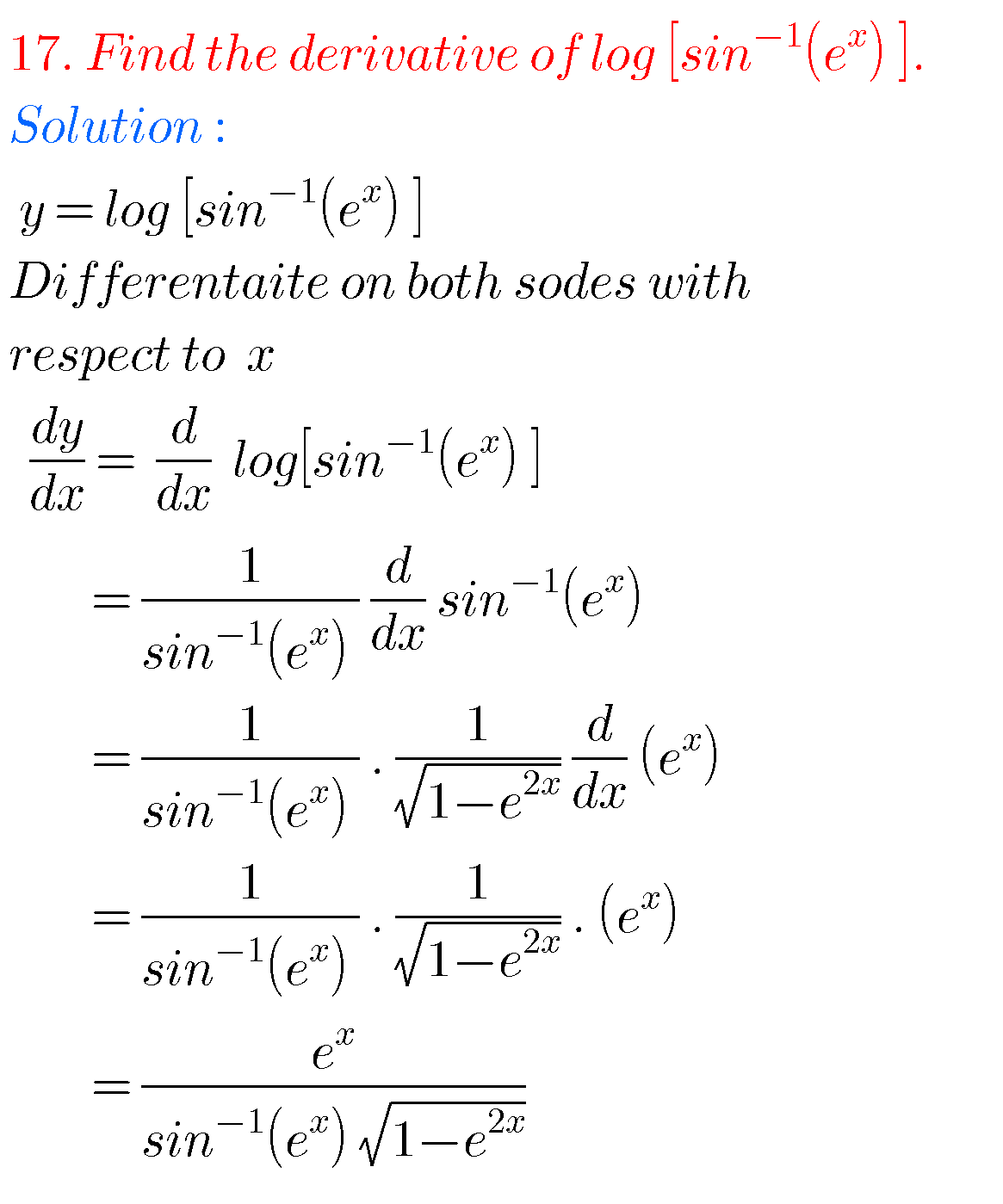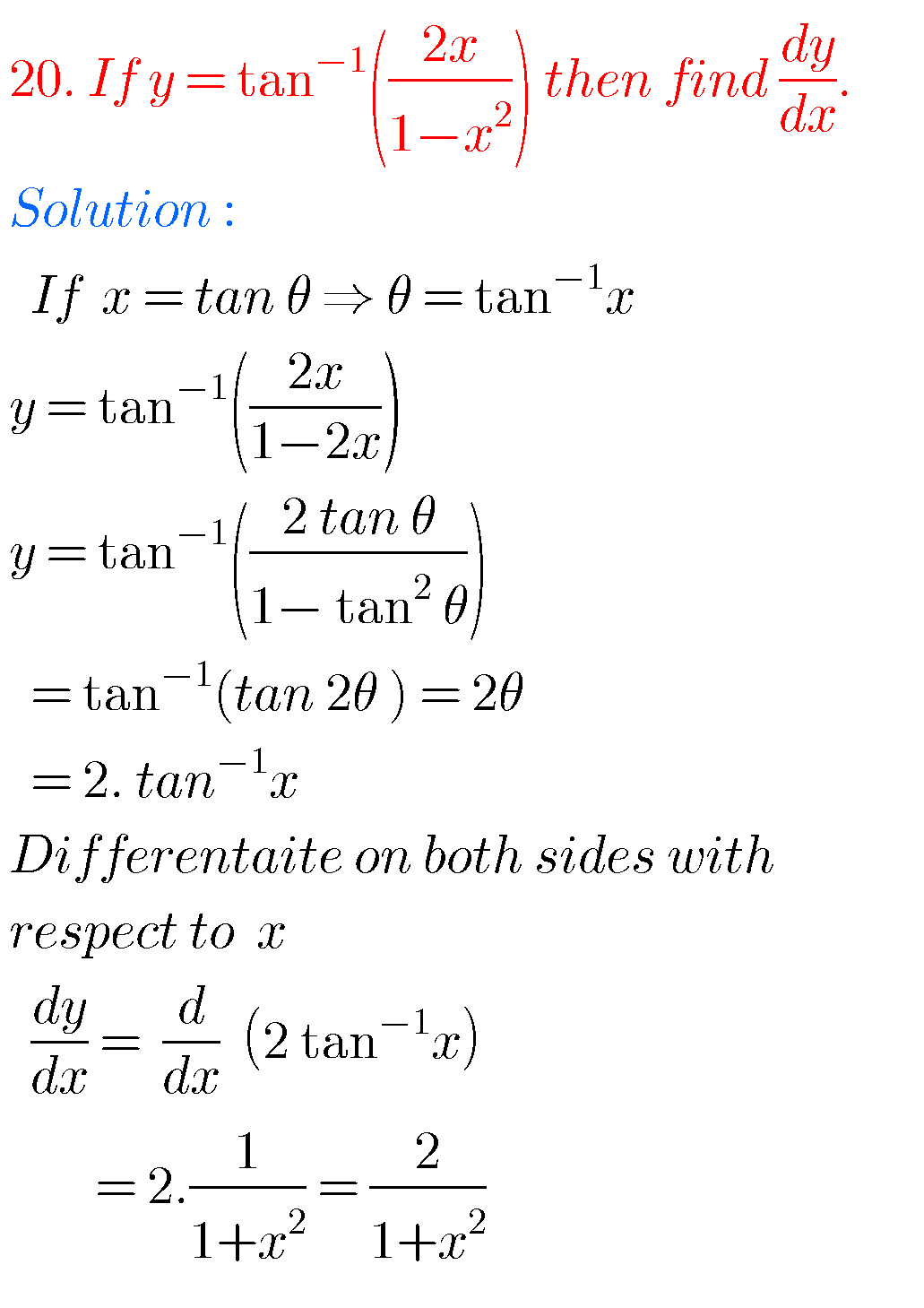###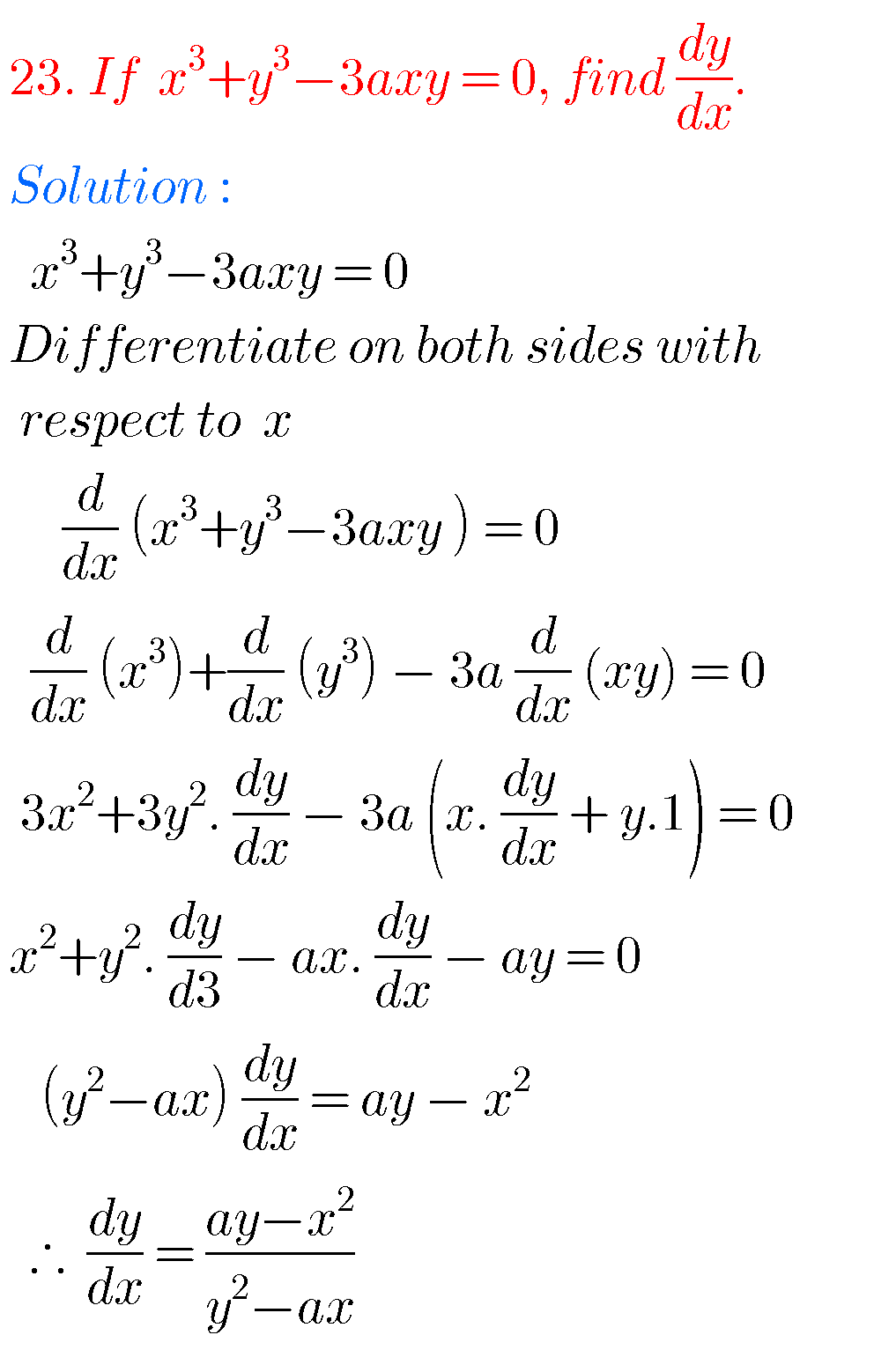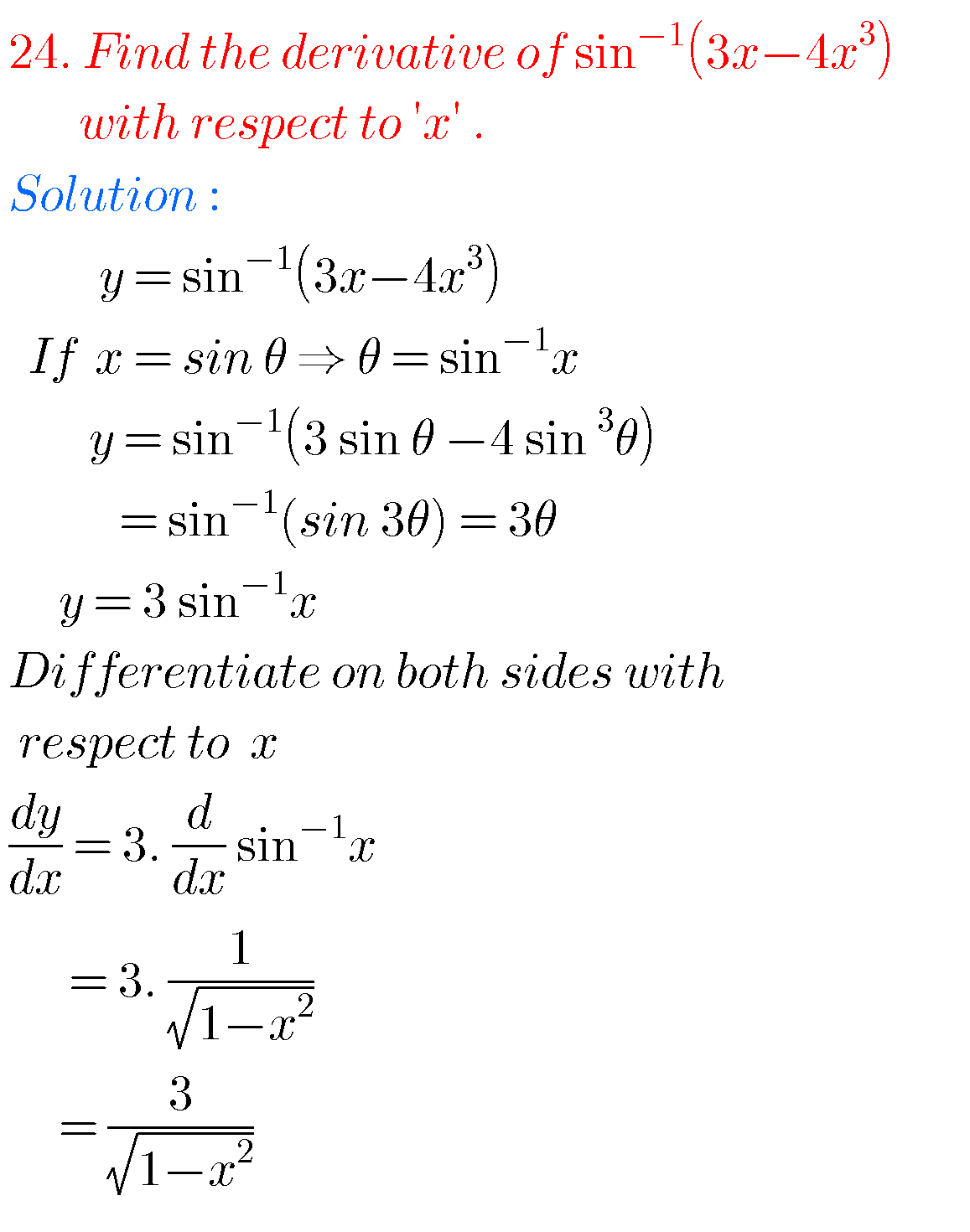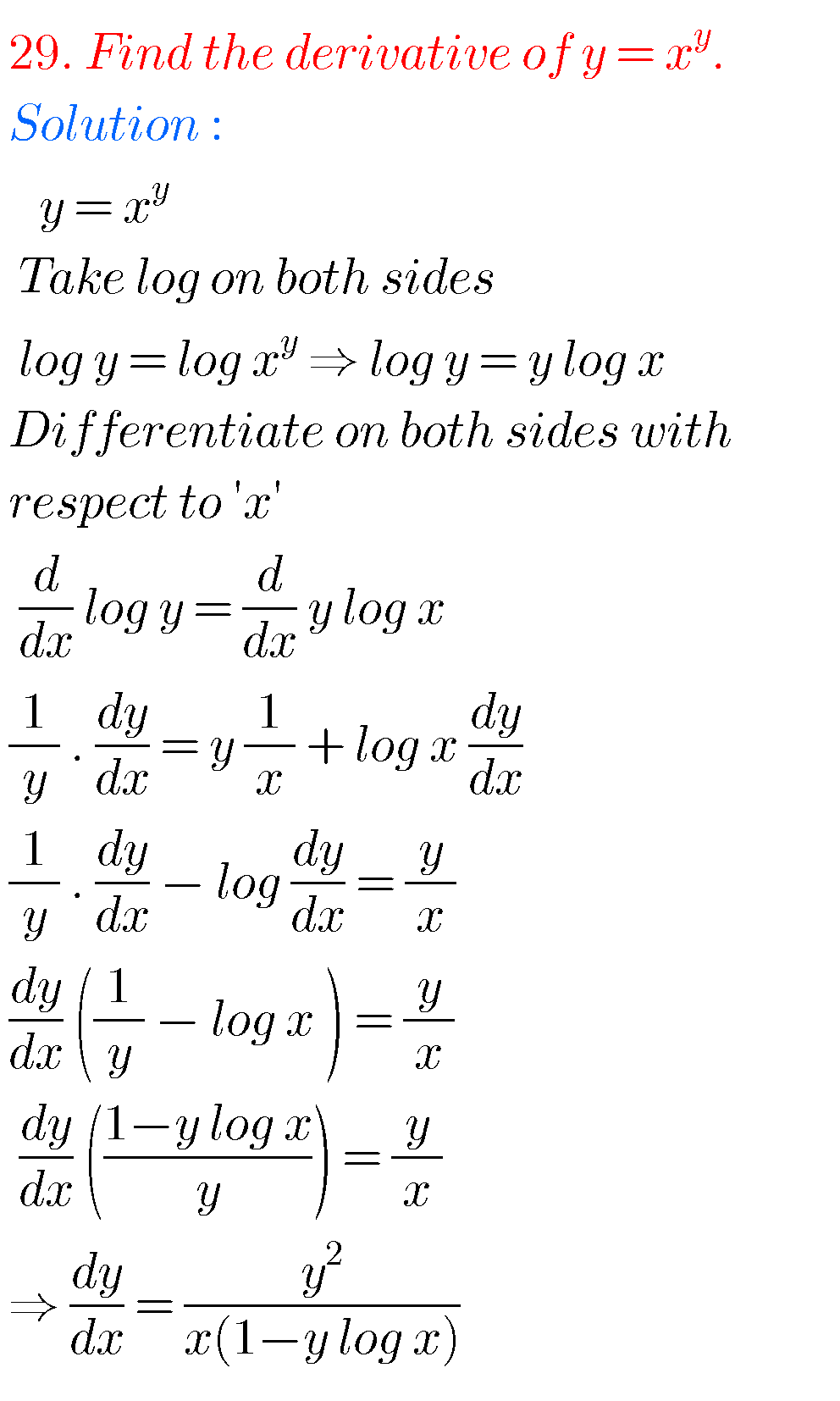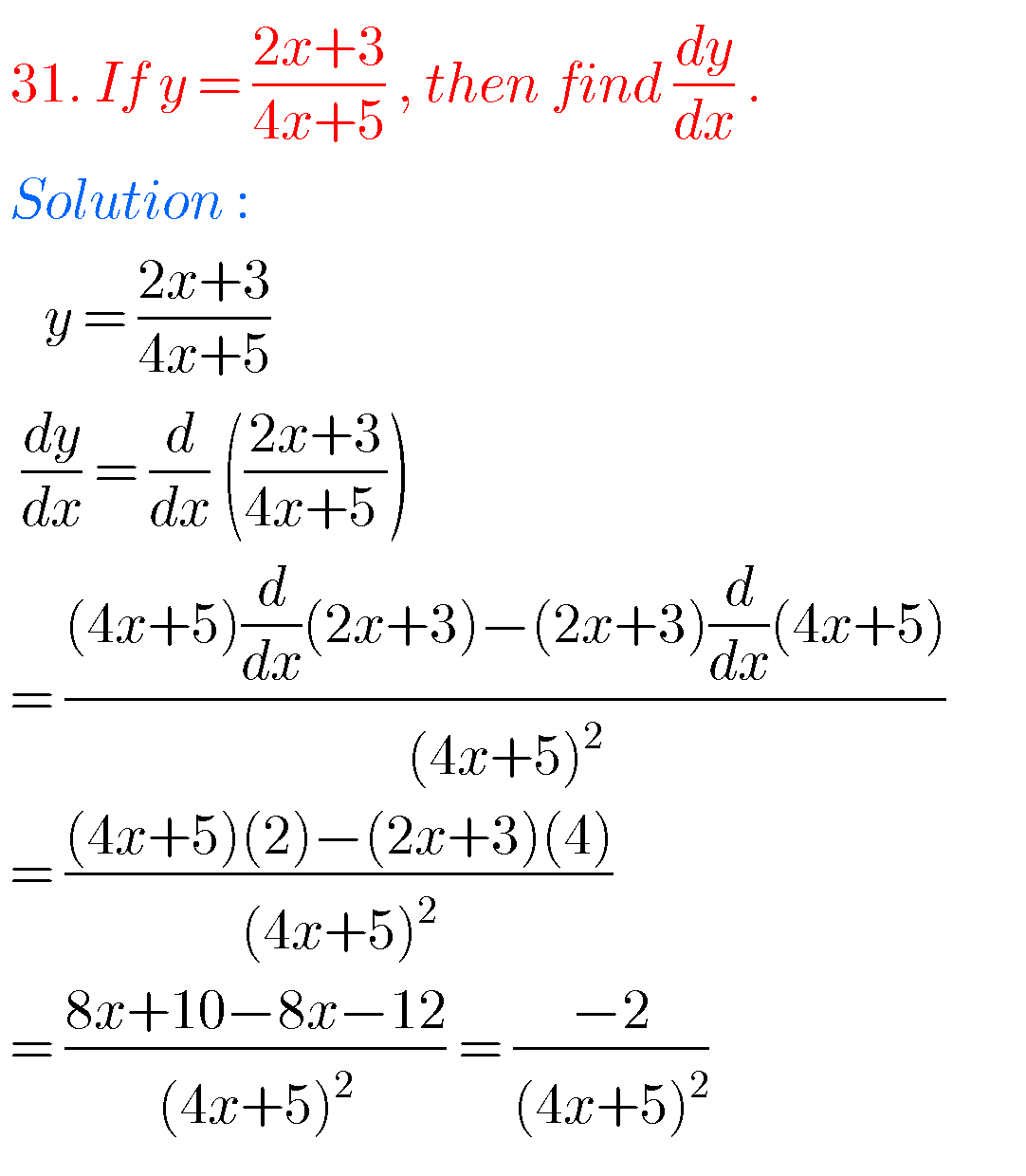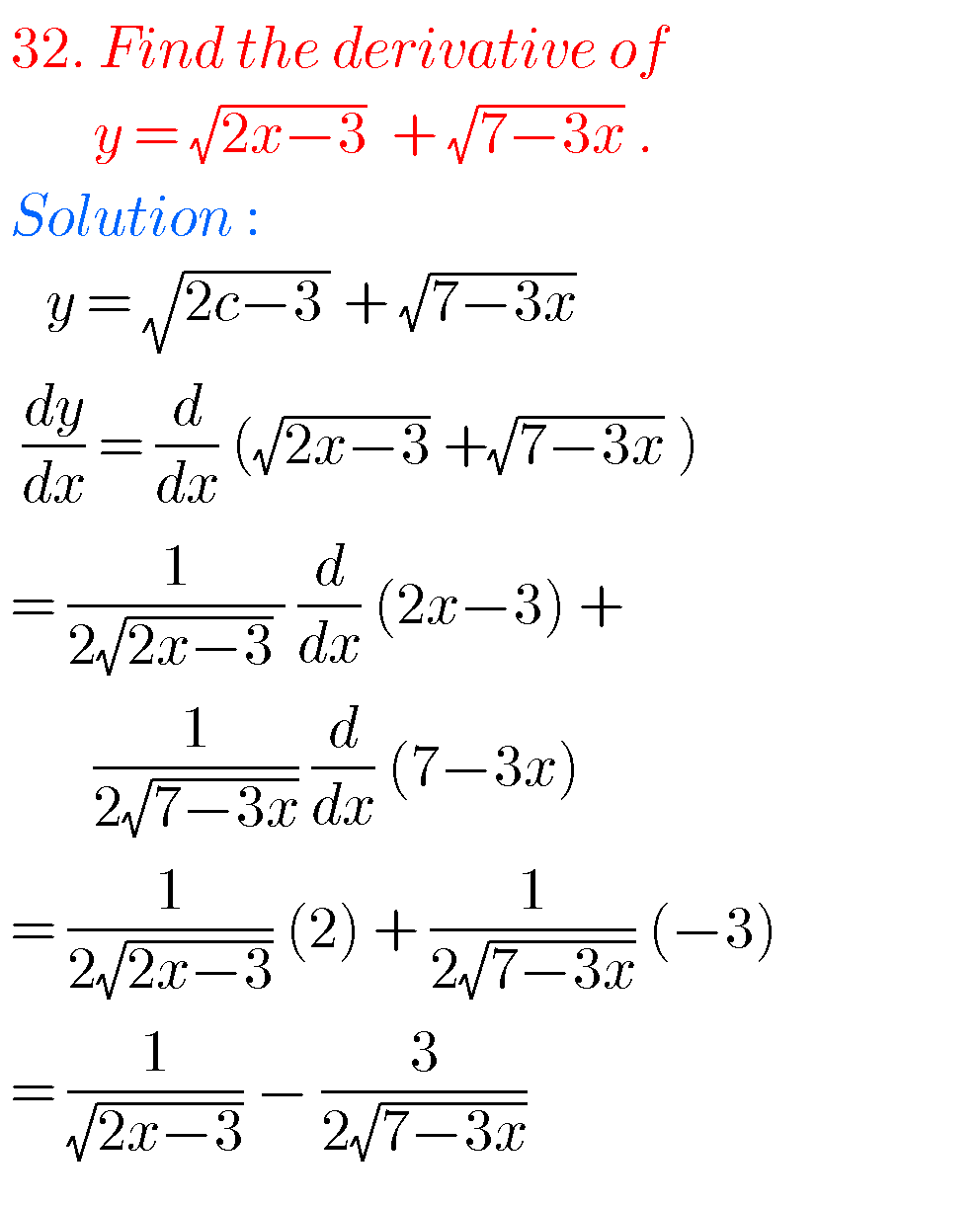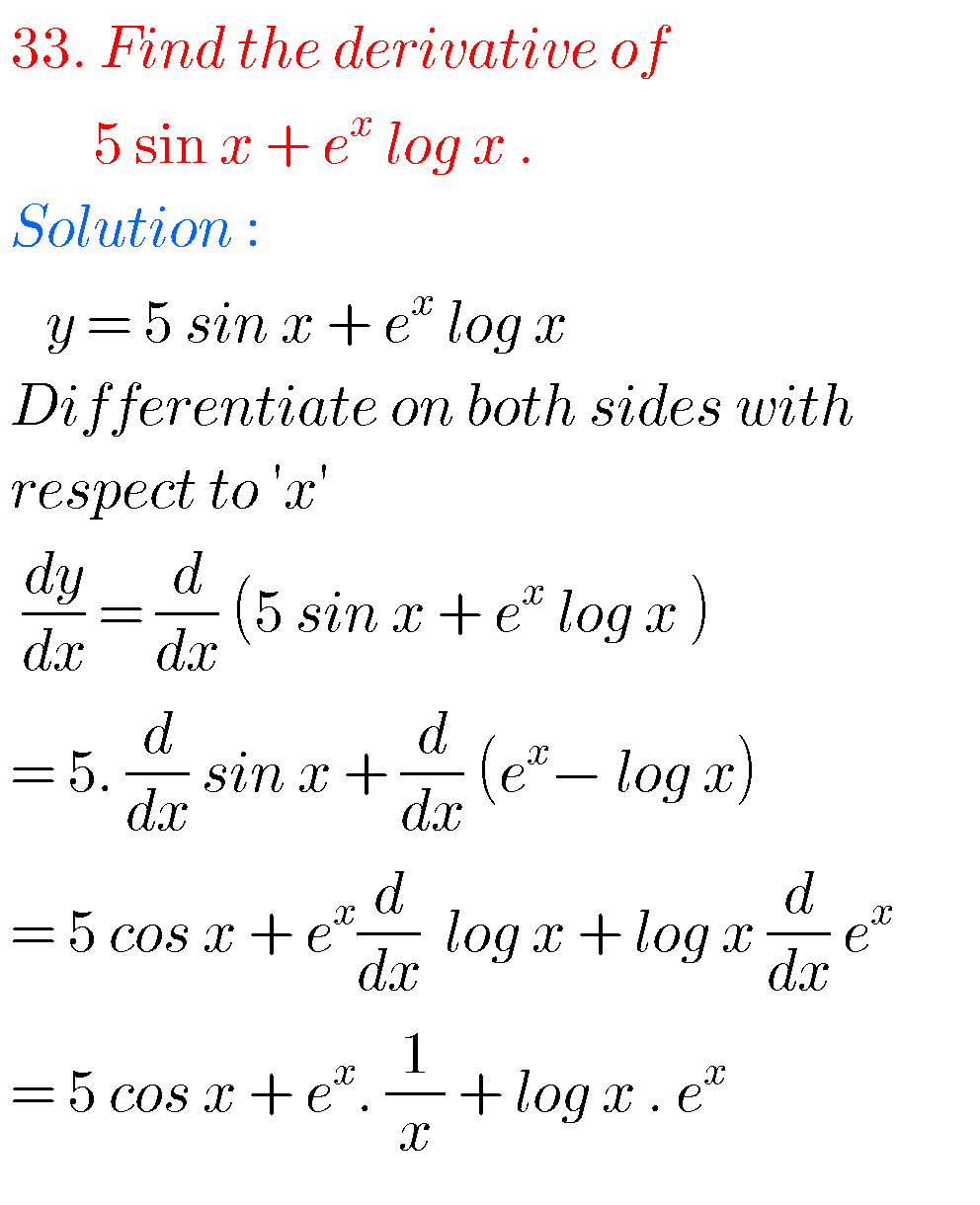### Differentiation inter first year solutions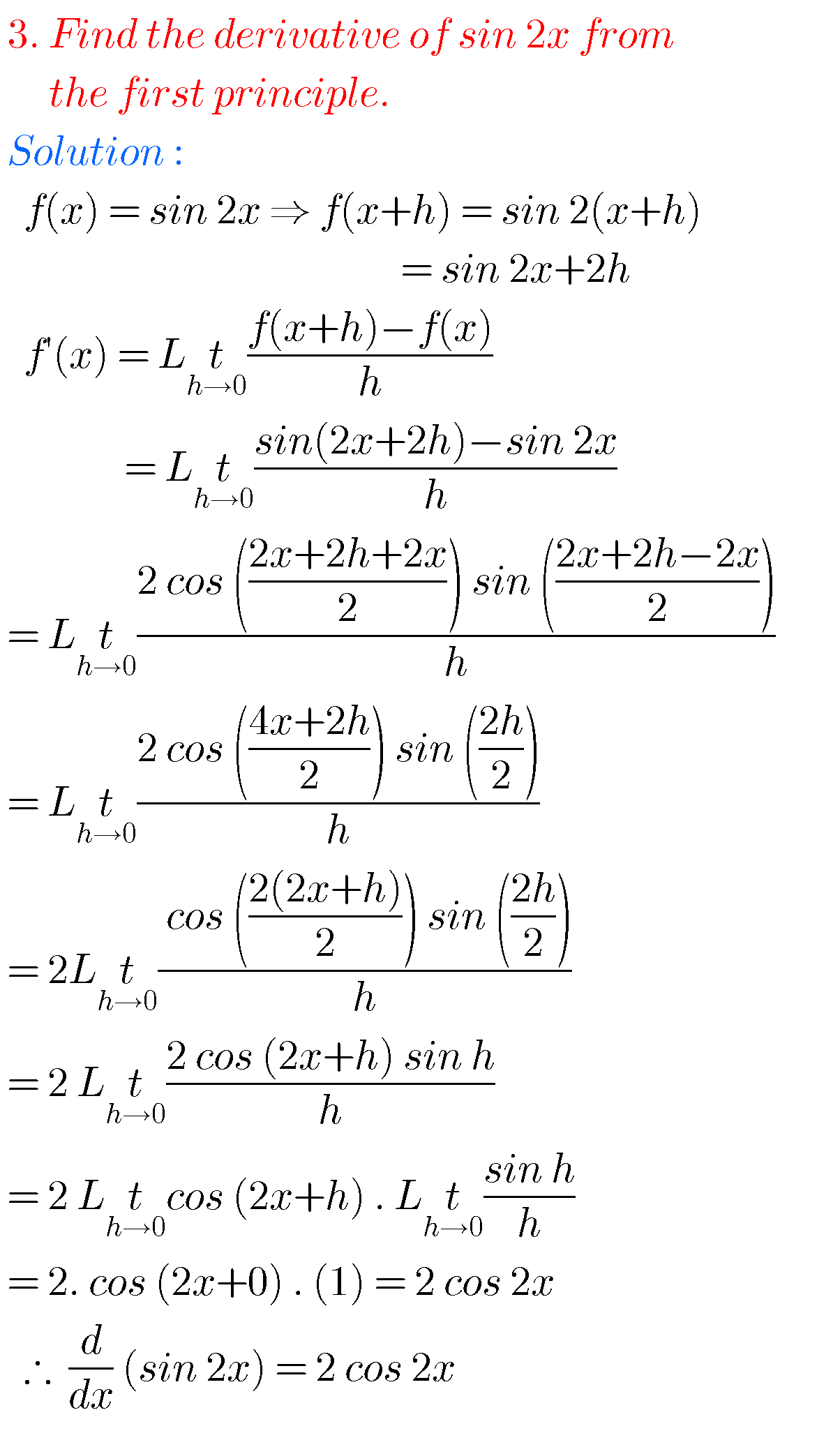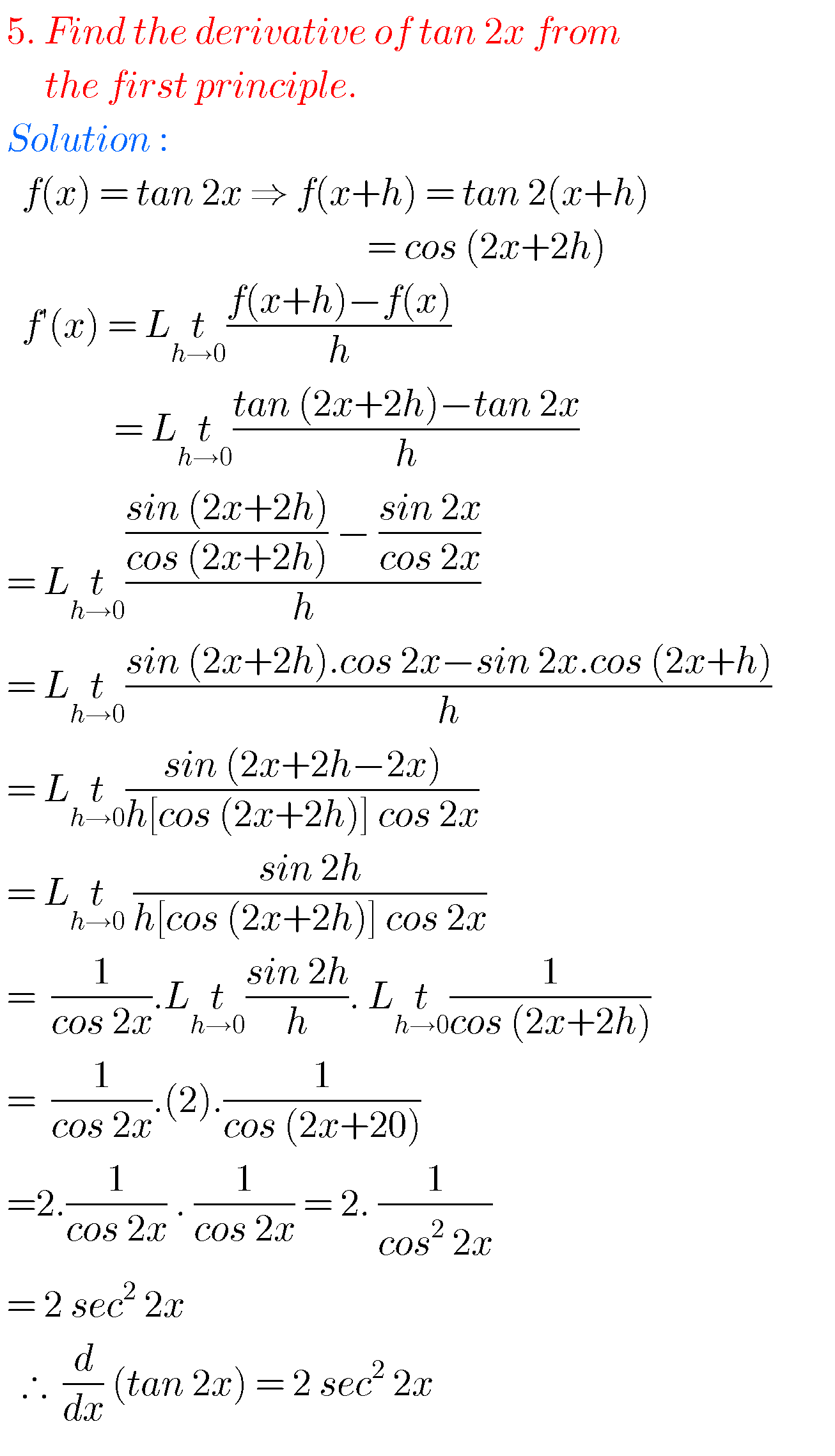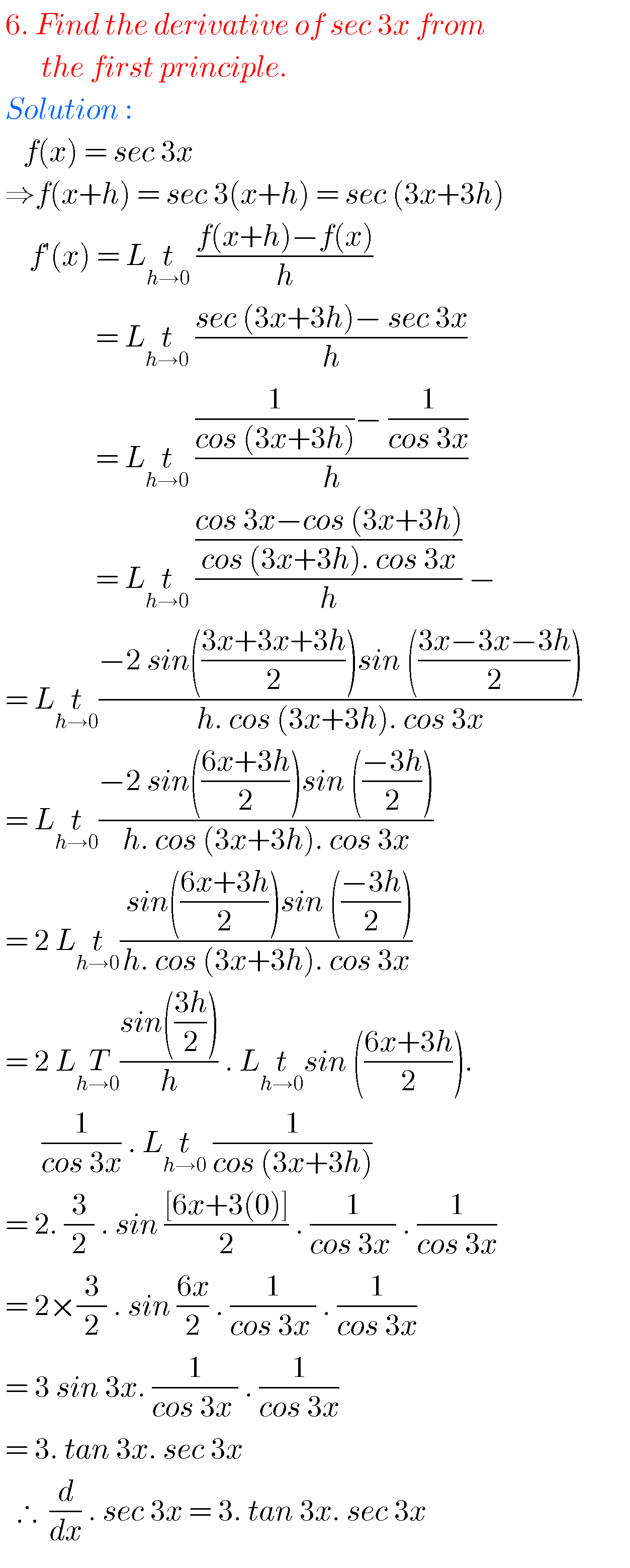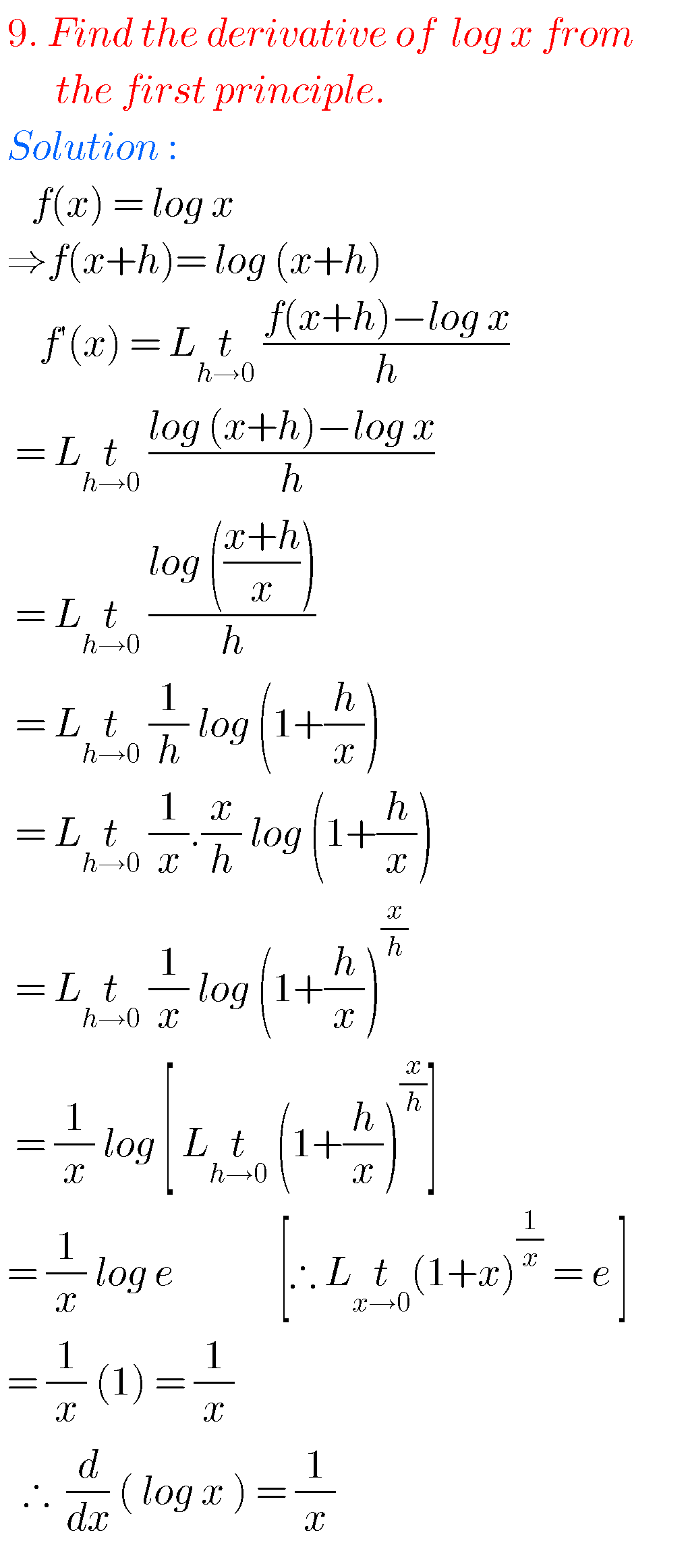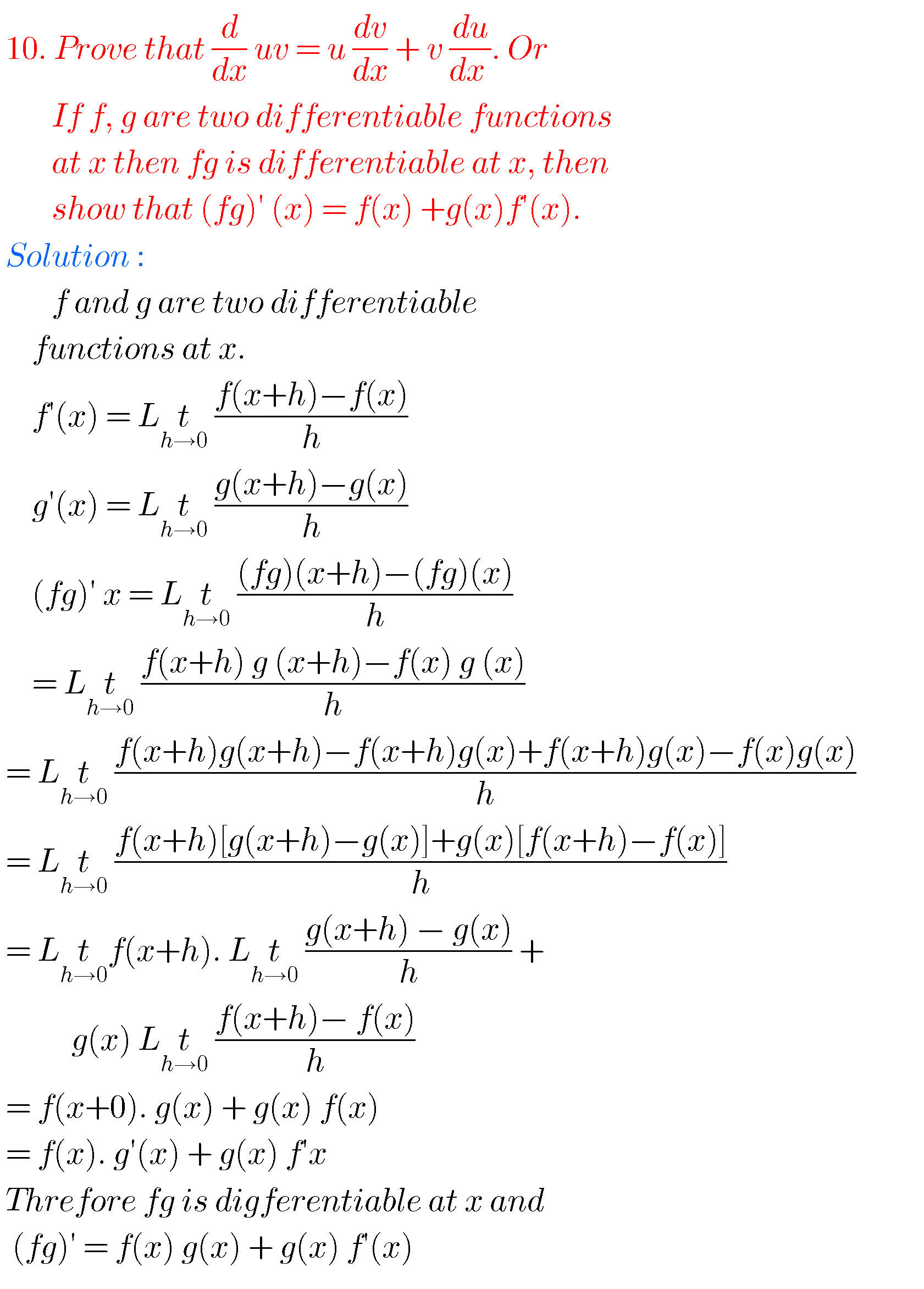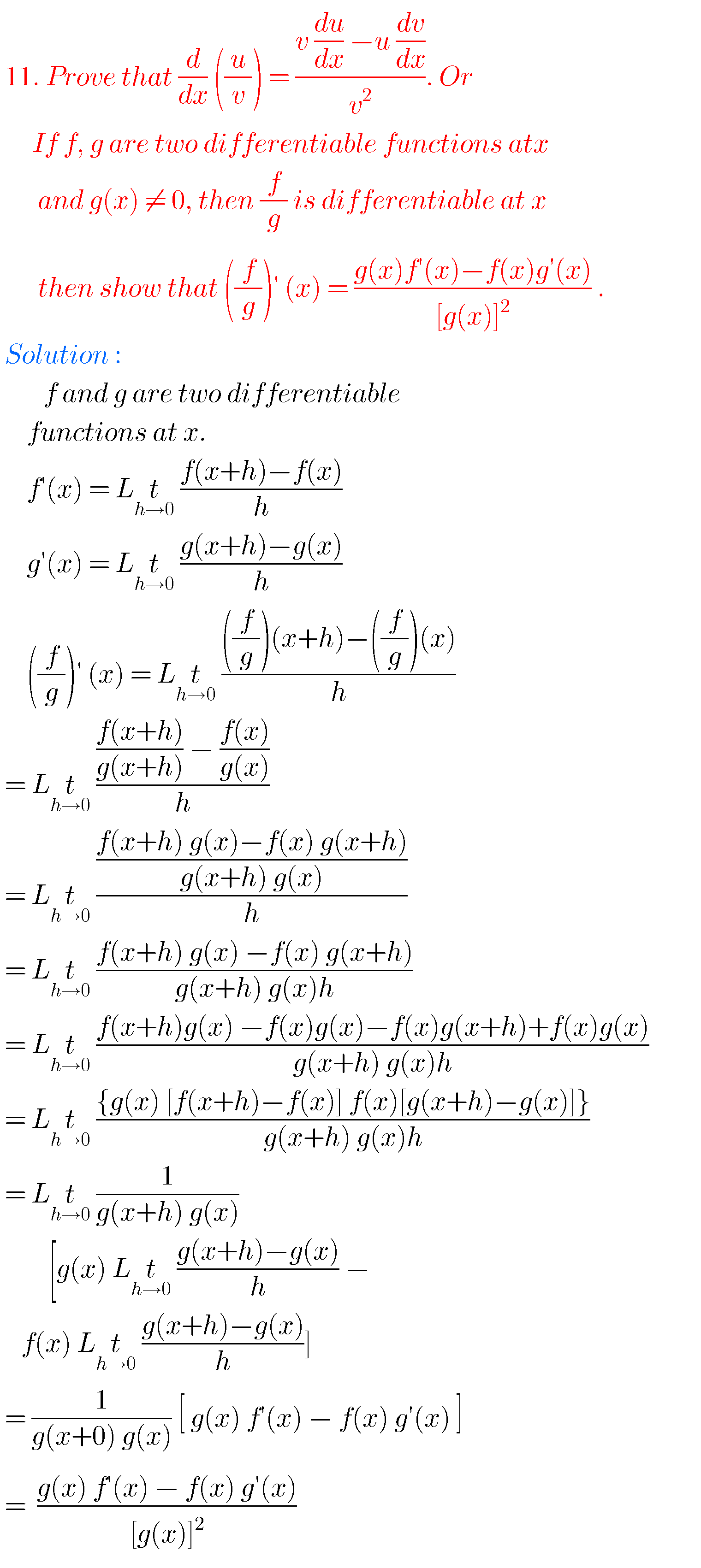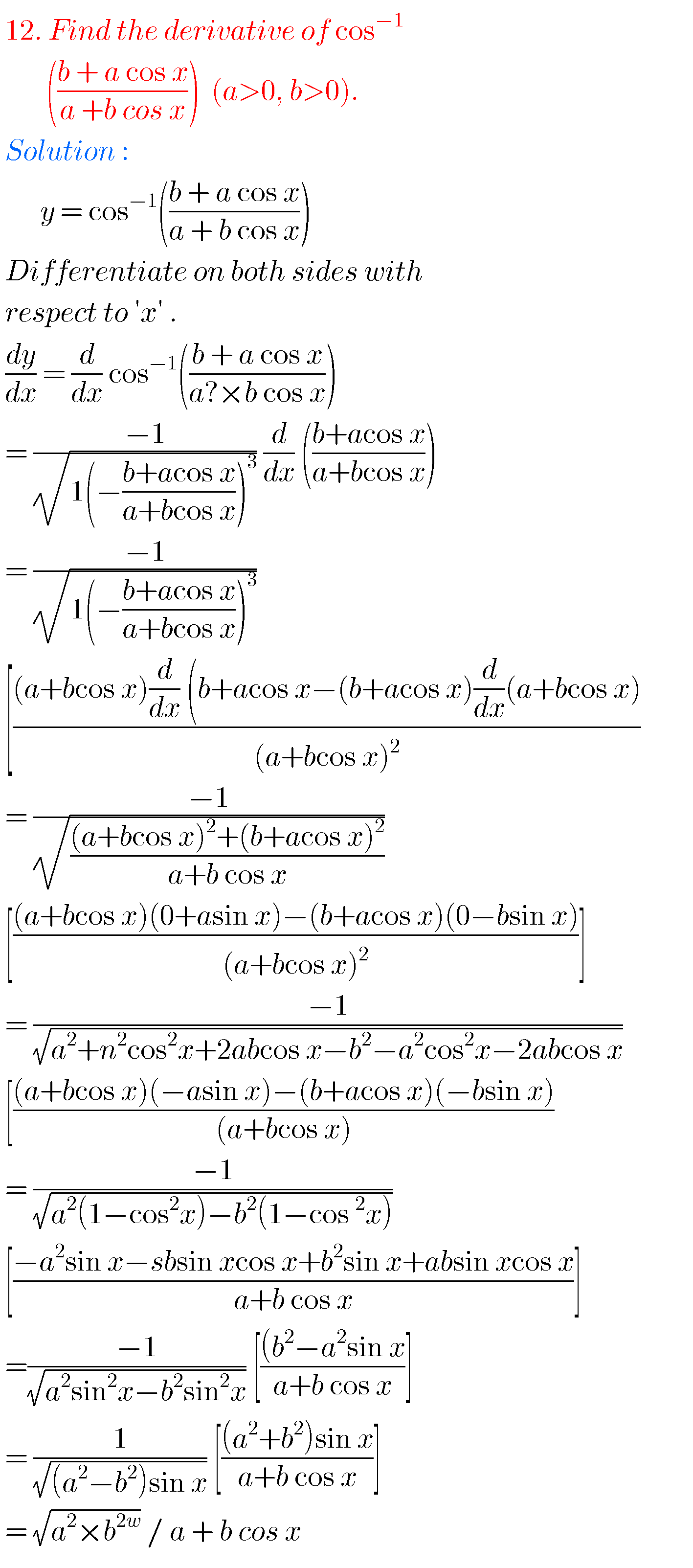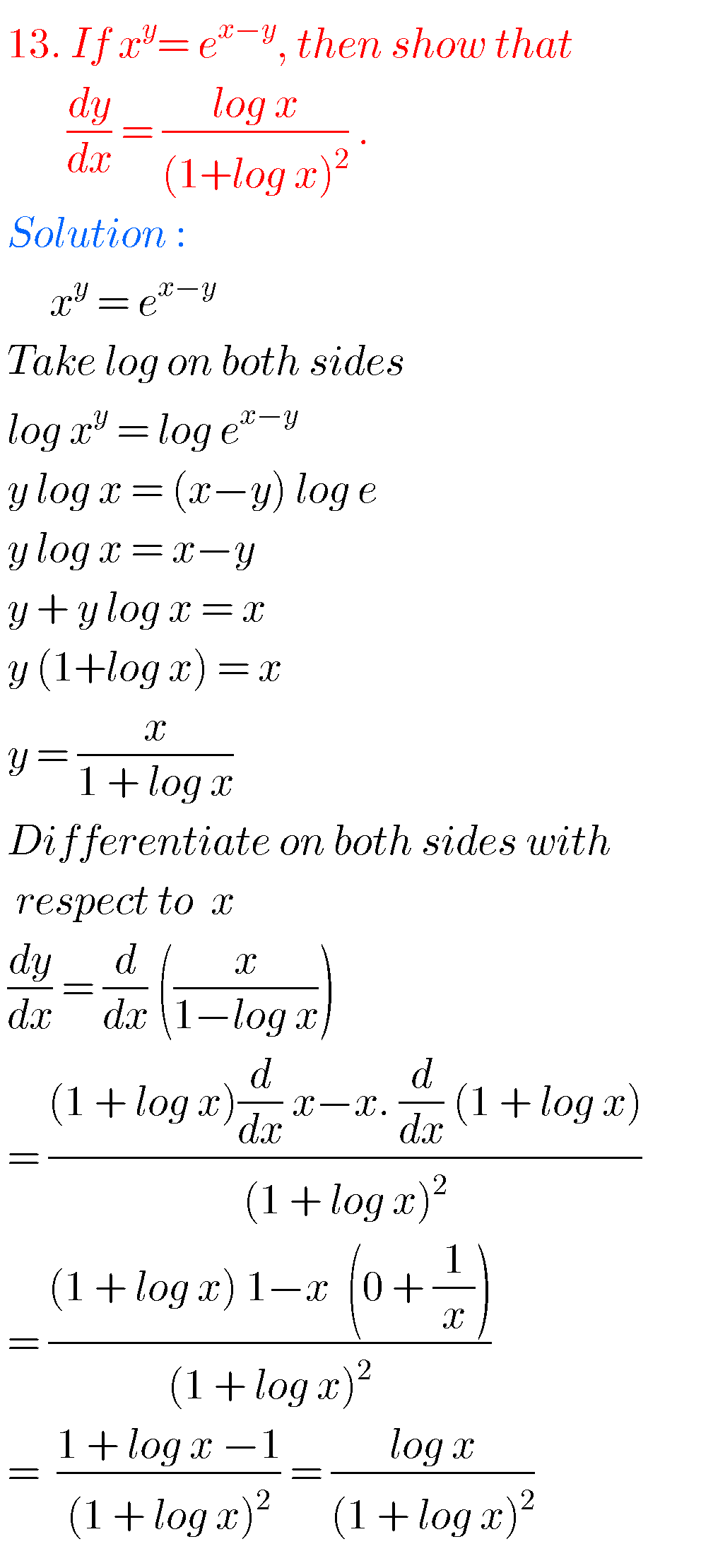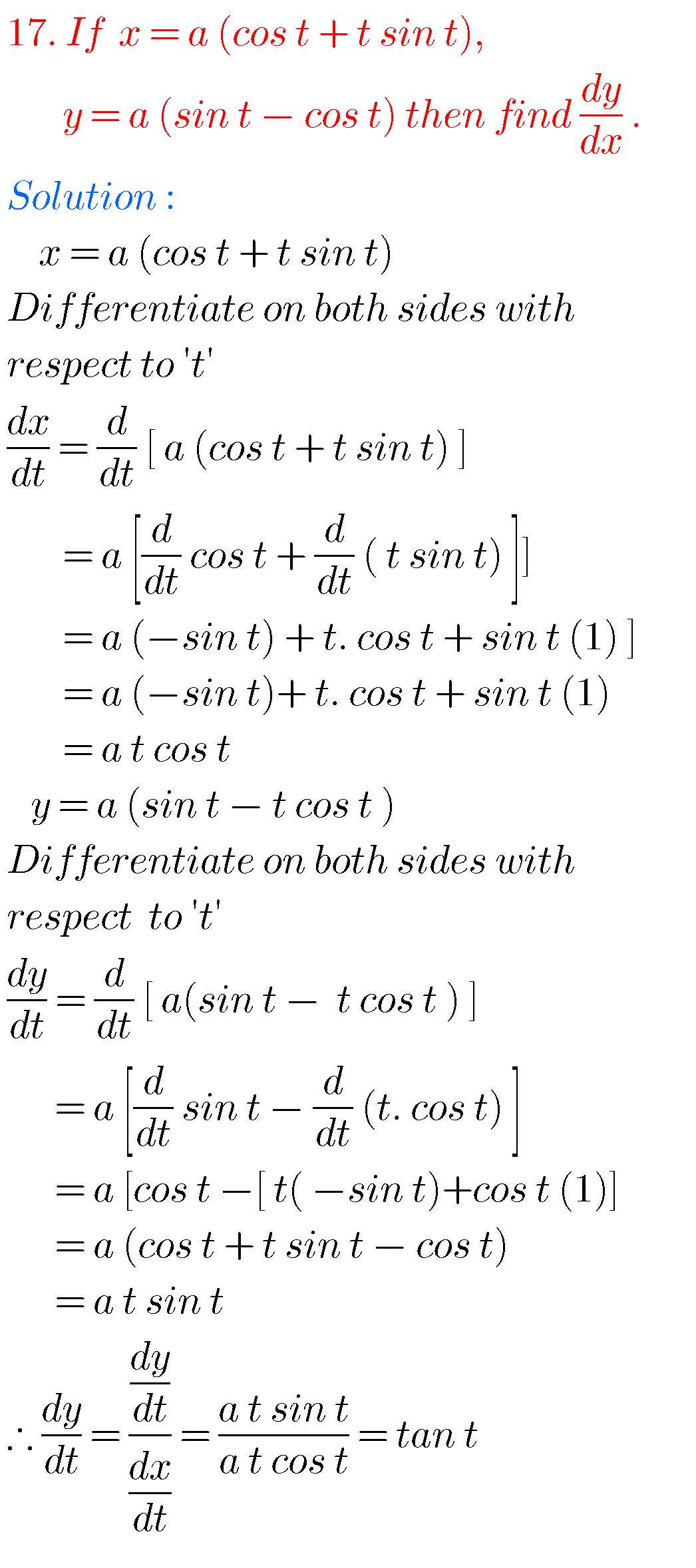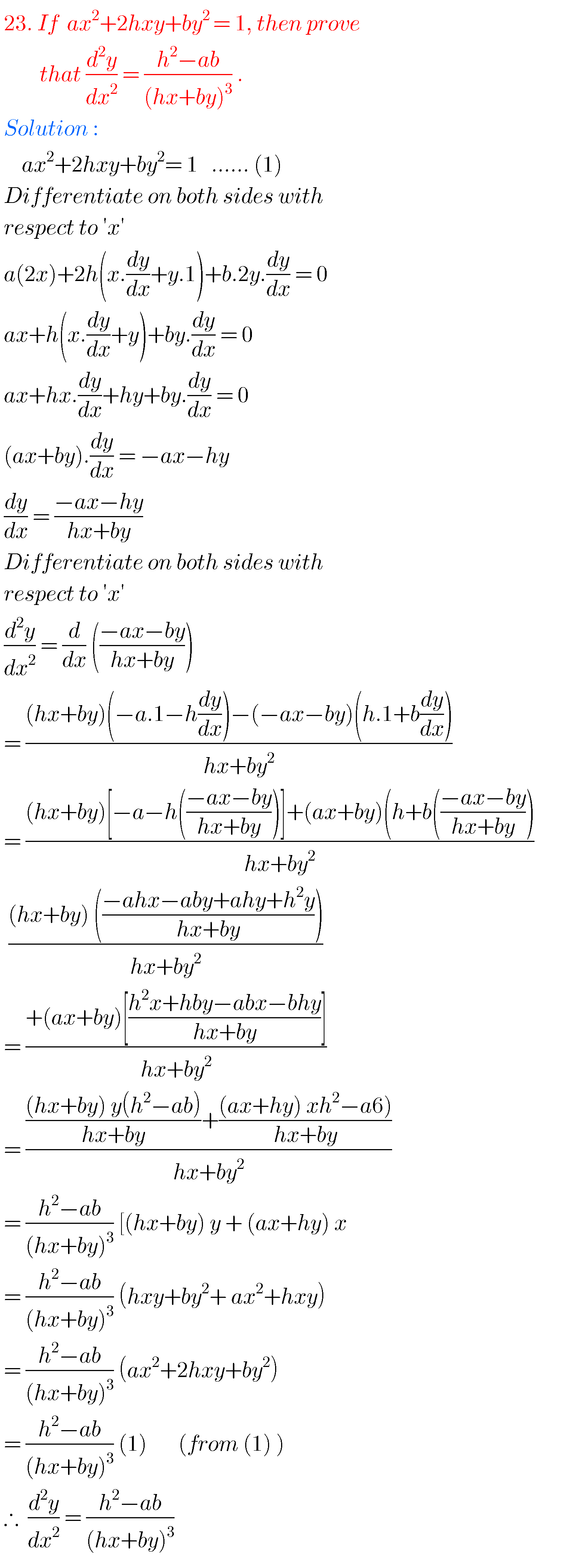### Differentiation inter solutions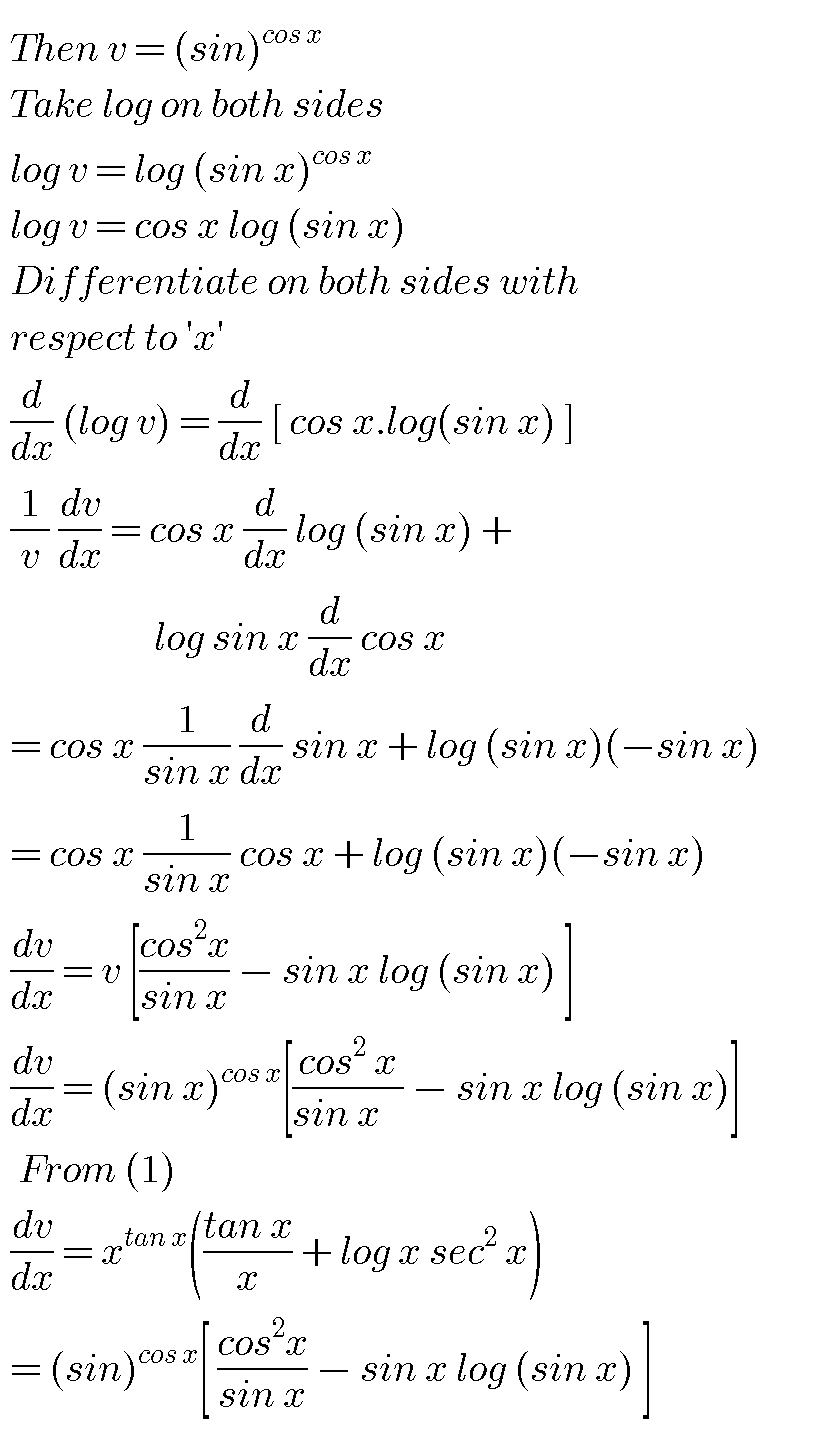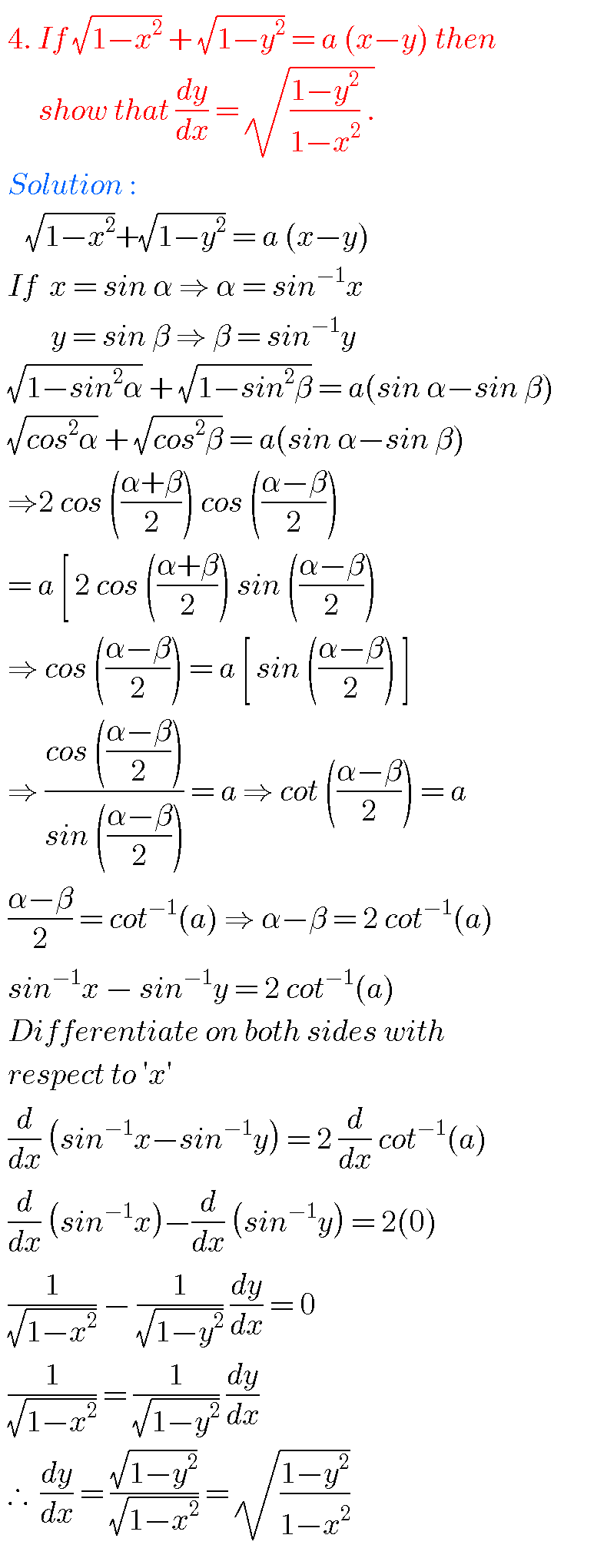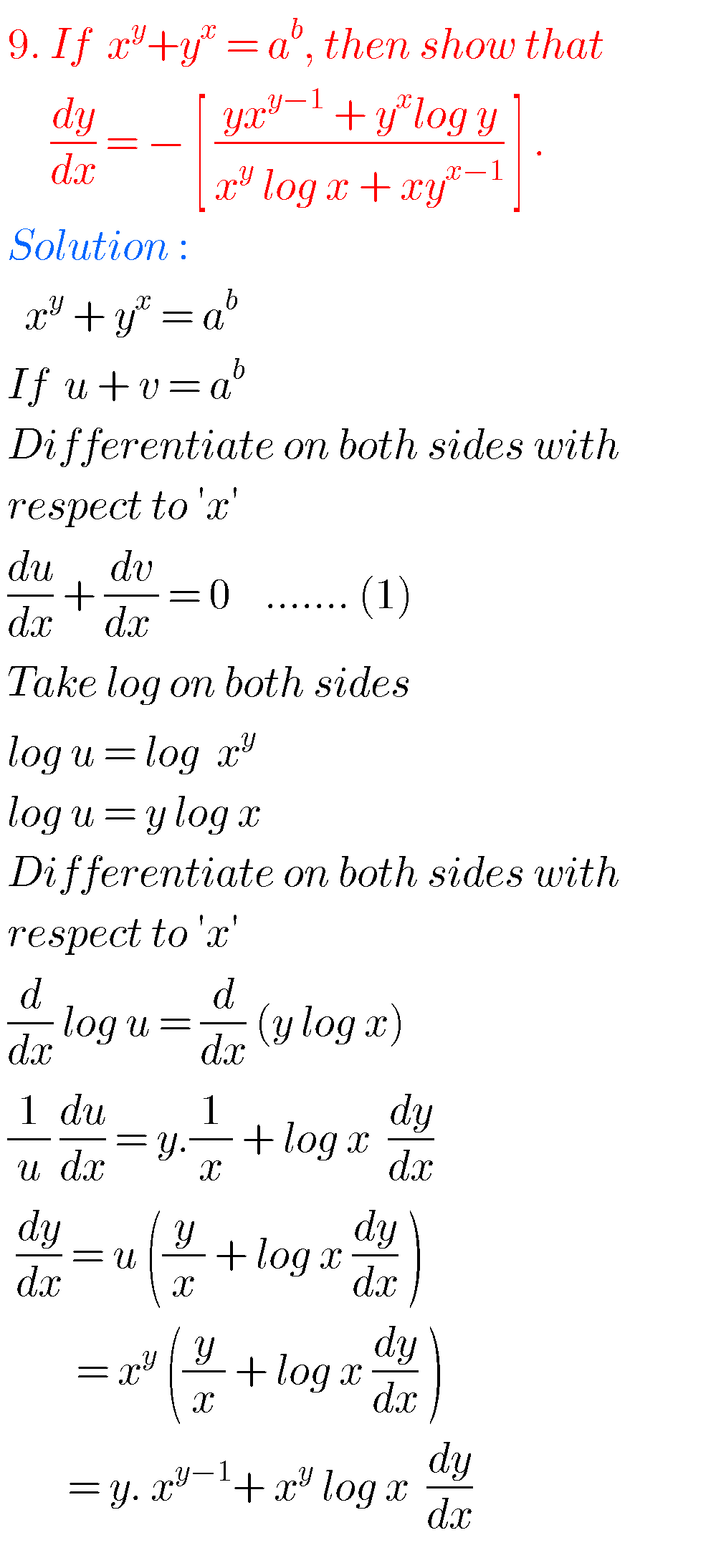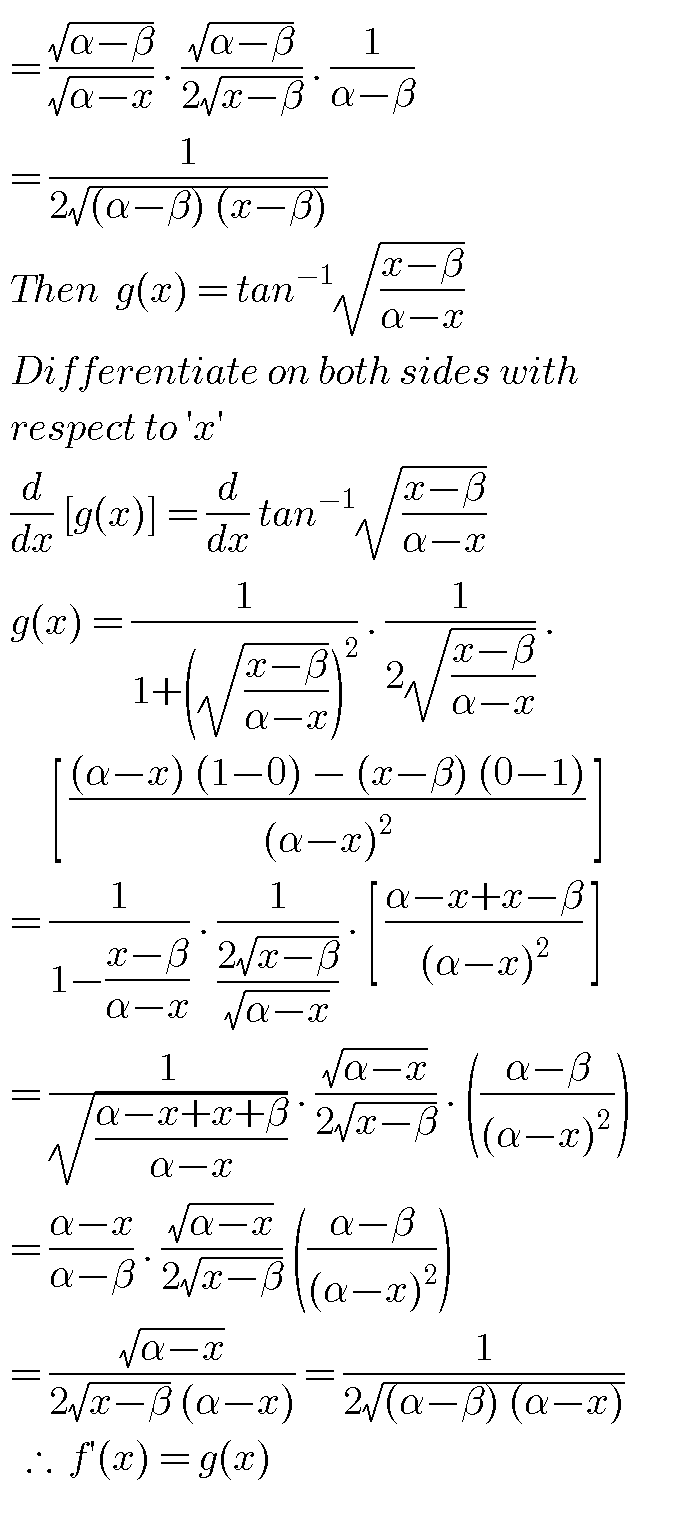Note : Observe the solutions and try them in your own methods.

Maths real numbers solutions class 10

You can see solutions for Inter Maths IIB

1. Circle

3. Parabola

4. Ellipse

You can also see solutions for Inter Maths IIA

For examination purpose you can see

Complex numbers

De Moivre’ s Theorem